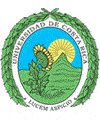``` Universidad de Costa Rica Escuela de Ciencias de la Computación e Informática ```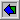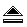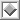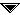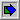# `BinarySearch()`

 ```procedure BINSRCH(F,n,i,K) // Search an ordered sequential file F with records R1, ...,Rn and the keys K1 ≤ K2 ≤ ... ≤ Kn for a record Ri such that Ki = K; i = 0 if there is no such record else Ki = K. Throughout the algorithm, l is the smallest index such that Kl may be K and u the largest index such that Ku may be K// l ← 1; u ← n while l ≤ u do m ← ë(l + u)/2ű //compute index of middle record// case :K > Km: l ← m + 1 //look in upper half// :K = Km: i ← m; return :K < Km: u ← m - 1 //look in lower half// end end i ← 0 //no record with key K// end BINSRCH ```
Horowitz, E.; Sahni, S.
"Fundamentals of Data Structures"; Computer Science Press; 1982.